# ShanaEncoder 2pass中的慢速一通道（fastfirstpass）有什么作用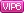成长值: 25675

[LV.Master]伴坛终老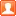发表于 2020/2/17 22:37 | 显示全部楼层 |阅读模式 |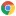Google Chrome 80.0.3987.106|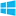Windows 10

 -fastfirstpass 0 表示开启慢速一通道 慢速一通道，代表，1pass阶段，使用相同的预设，例如设置slower，那么1pass就会走相同的slower -fastfirstpass 1 或者不存在则表示开启快速一通道 快速一通道，代表，1pass阶段，使用superfast预设进行快速的1pass过程 开启慢速一通道参数，值为slower的me=umh，1pass过程速度为18fps cabac=1 ref=8 deblock=1:0:0 analyse=0x3:0x133 me=umh subme=9 psy=0 mixed_ref=1 me_range=16 chroma_me=1 trellis=2 8x8dct=1 cqm=0 deadzone=21,11 fast_pskip=1 chroma_qp_offset=0 threads=9 lookahead_threads=1 sliced_threads=0 nr=0 decimate=1 interlaced=0 bluray_compat=0 constrained_intra=0 bframes=3 b_pyramid=2 b_adapt=2 b_bias=0 direct=3 weightb=1 open_gop=0 weightp=2 keyint=600 keyint_min=60 scenecut=40 intra_refresh=0 rc_lookahead=60 rc=abr mbtree=1 bitrate=6000 ratetol=1.0 qcomp=0.60 qpmin=0 qpmax=69 qpstep=4 vbv_maxrate=24000 vbv_bufsize=3000 nal_hrd=none filler=0 ip_ratio=1.40 aq=2:1.00 关闭慢速一通道参数，值为superfast的me=dia，1pass过程速度为72fps cabac=1 ref=1 deblock=1:0:0 analyse=0x1:0 me=dia subme=2 psy=0 mixed_ref=0 me_range=16 chroma_me=1 trellis=0 8x8dct=0 cqm=0 deadzone=21,11 fast_pskip=1 chroma_qp_offset=0 threads=9 lookahead_threads=4 sliced_threads=0 nr=0 decimate=1 interlaced=0 bluray_compat=0 constrained_intra=0 bframes=3 b_pyramid=2 b_adapt=2 b_bias=0 direct=3 weightb=1 open_gop=0 weightp=2 keyint=600 keyint_min=60 scenecut=40 intra_refresh=0 rc_lookahead=60 rc=abr mbtree=1 bitrate=6000 ratetol=1.0 qcomp=0.60 qpmin=0 qpmax=69 qpstep=4 vbv_maxrate=24000 vbv_bufsize=3000 nal_hrd=none filler=0 ip_ratio=1.40 aq=2:1.00 比如使用slower预设开启慢速一通道就明显了，1pass过程速度会差距很大 效果对比，其实不算太明显，基本没区别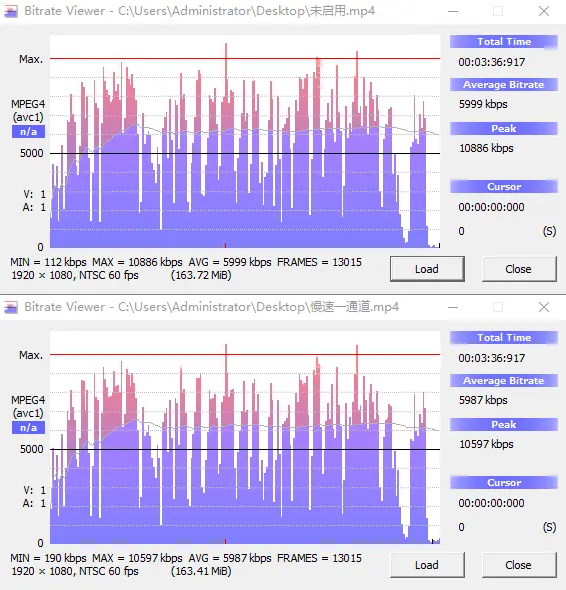想要开启2pass并且不缺时间压制的话，建议勾选慢速一通道

[LV.10]以坛为家III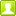发表于 2020/2/18 01:06 | 显示全部楼层 |Google Chrome 79.0.3945.130|Windows 10[LV.3]偶尔看看II发表于 2020/3/22 11:42 | 显示全部楼层 |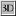uBrowser 6.2.4098.3|Windows 10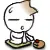本版积分规则 回帖后跳转到最后一页

GMT+8, 2020/9/19 13:40 , Processed in 0.151953 second(s), 22 queries , MemCache On.